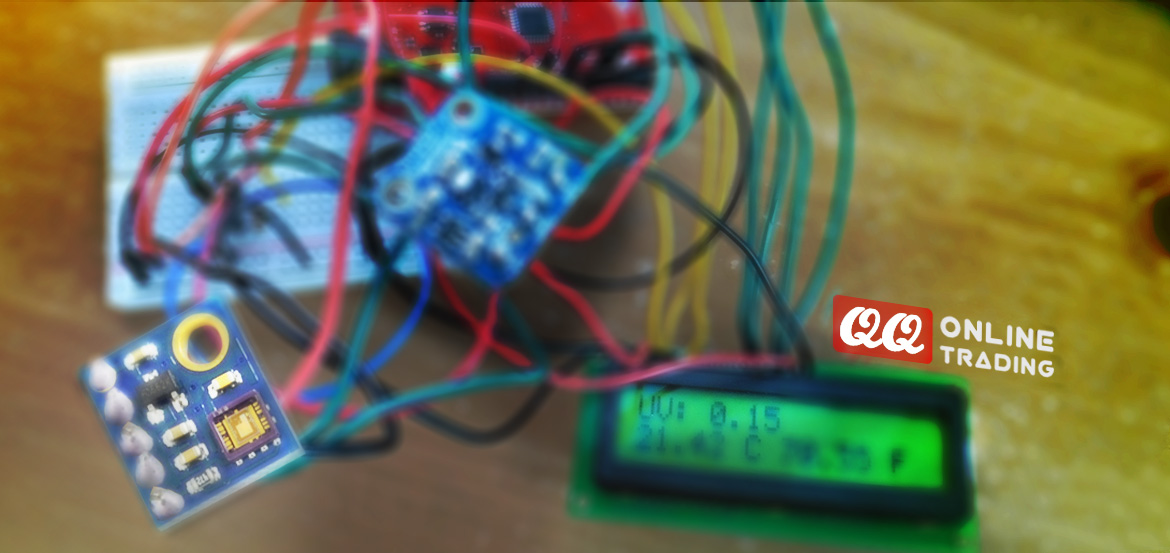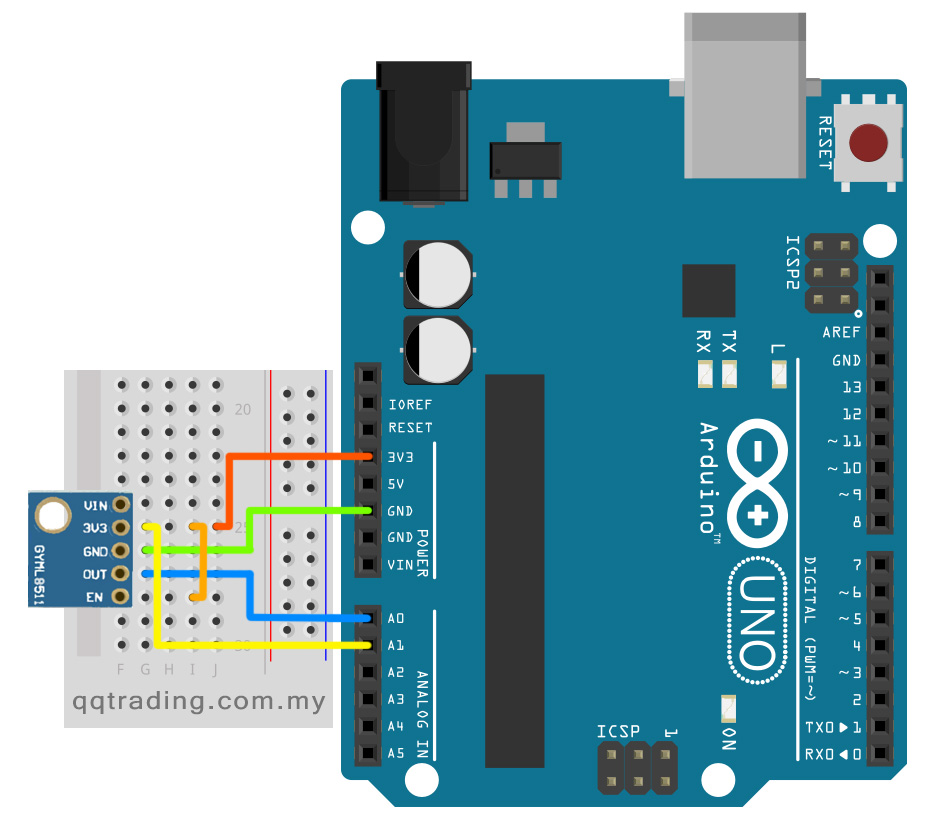# Ultraviolet Light UV Sensor Module w/ Amplifier (Wave-Length max. 365nm) GY-8511

• Was  RM40.00
• ## RM30.00

• Product Code: GY-8511
• Availability: In StockGY-8511 (a.k.a ML8511) Ultraviolet (UV) light sensor output an analog signal by amount of UV light that’s detected. This breakout can be very handy in creating devices that warn the user of sunburn or detect the UV index as it relates to weather conditions.

This sensor detects 280-365nm light most effectively. This is categorized as part of the UVB (burning rays) spectrum and most of the UVA (tanning rays) spectrum. It outputs an analog voltage that is linearly related to the measured UV intensity (mW/cm2). If your microcontroller can do an analog to digital signal conversion then you can detect the level of UV!

Features

• Optical sensor to UV-A and UV-B
• Realize equalization of an output voltage by trimming
• The analog voltage output proportional to UV light intensity
• Low current operation ( 300µA Typ.)  and low standby current ( 0.1µA Typ.)

Specifications

• Operating voltage: 2.7 to 3.6V DC
• Operating temperature: -20 to +70°C
• Maximum-sensitivity wave length: 365nm
• Sensitivity region: UV-A and UV-B
• Output type: Analog
• Current Consumption (Operating mode / Standby mode): 300µA / 0.1µA
• Dimensions: 1.3 x 1.1 x 0.2 cm

### Connect to ArduinoConnect the following ML8511 breakout board to Arduino:

3.3V = 3.3V
OUT = A0
GND = GND
EN = 3.3V
3.3V = A1

These last two connections are a little different. Connect the EN pin on the breakout to 3.3V on the breakout.
This will enable the output. Also connect the 3.3V pin of the breakout to Arduino pin 1.

```//Hardware pin definitions
int UVOUT = A0; //Output from the sensor
int REF_3V3 = A1; //3.3V power on the Arduino board

void setup()
{
Serial.begin(9600);

pinMode(UVOUT, INPUT);
pinMode(REF_3V3, INPUT);

Serial.println("ML8511 example");
}

void loop()
{

//Use the 3.3V power pin as a reference to get a very accurate output value from sensor
float outputVoltage = 3.3 / refLevel * uvLevel;

float uvIntensity = mapfloat(outputVoltage, 0.99, 2.8, 0.0, 15.0); //Convert the voltage to a UV intensity level

Serial.print("output: ");
Serial.print(refLevel);

Serial.print("ML8511 output: ");
Serial.print(uvLevel);

Serial.print(" / ML8511 voltage: ");
Serial.print(outputVoltage);

Serial.print(" / UV Intensity (mW/cm^2): ");
Serial.print(uvIntensity);

Serial.println();

delay(100);
}

//Takes an average of readings on a given pin
//Returns the average
{
unsigned int runningValue = 0;

for(int x = 0 ; x < numberOfReadings ; x++)

return(runningValue);
}

//The Arduino Map function but for floats
//From: http://forum.arduino.cc/index.php?topic=3922.0
float mapfloat(float x, float in_min, float in_max, float out_min, float out_max)
{
return (x - in_min) * (out_max - out_min) / (in_max - in_min) + out_min;
}```

## Write a review

Note: HTML is not translated!